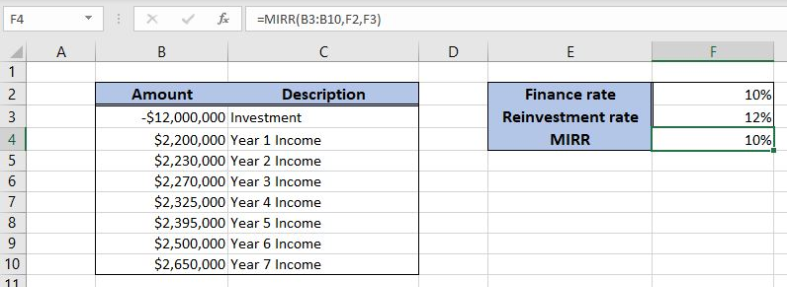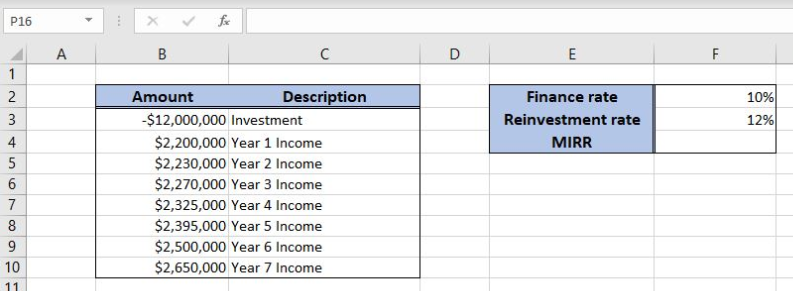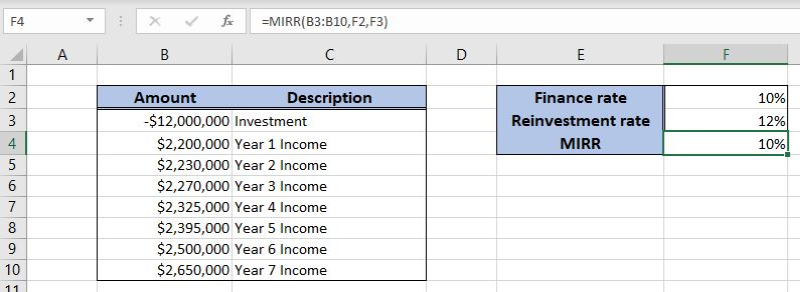Get instant live expert help with Excel or Google Sheets“My Excelchat expert helped me in less than 20 minutes, saving me what would have been 5 hours of work!”

#### Post your problem and you’ll get expert help in seconds.

Your message must be at least 40 characters
Our professional experts are available now. Your privacy is guaranteed.

# How to Find the Modified Internal Rate of Return in Excel

Excel allows a user to get a modified internal rate of return of an investment using the MIRR function. This function includes a discount rate and reinvestment rate in the calculation. This step by step tutorial will assist all levels of Excel users in finding a modified internal rate of return in ExcelFigure 1. The result of the MIRR function

## Syntax of the MIRR Formula

The generic formula for the MIRR function is:

`=MIRR (values, [guess])`

The parameter of the MIRR function is:

• values – a range of cells containing values, including initial investment and incomes. The investment must have negative sign, as it is a cost
• finance_rate – a discount rate of the investment returns
• reinvest_rate – an interest rate for reinvested returns.

## Setting up Our Data for the MIRR Function

Let’s look at the structure of the data we will use. In column B (“Amount”), we have values including initial investment and yearly incomes. In column C (“Description”) we have description of every amount. In the cell F2, we have finance rate, while in the cell F3 there is the reinvestment rate. Finally, we want to get the MIRR in F4.Figure 2. Data that we will use in the MIRR example

## Get a MIRR of Values Using the MIRR Function

In our example, we want to get the MIRR of the values in the range B3:B10. The finance rate is 10% (F2) and the reinvestment rate is 12% (F3). The result will be in the cell F4.

The formula looks like:

`=MIRR(B3:B10, F2, F3)`

The parameter values is the range B3:B10. The finance_rate is in F2, while the reinvest_rate is in F3.

To apply the MIRR function, we need to follow these steps:

• Select cell F4 and click on it
• Insert the formula: `=MIRR(B3:B10, F2, F3)`
• Press enter.Figure 3. Using the MIRR function to get the modified internal rate of the investment

Finally, the result in the cell F4 is 10%, which is the modified internal rate of the investment.

Most of the time, the problem you will need to solve will be more complex than a simple application of a formula or function. If you want to save hours of research and frustration, try our live Excelchat service! Our Excel Experts are available 24/7 to answer any Excel question you may have. We guarantee a connection within 30 seconds and a customized solution within 20 minutes.